What is excel formula?

Excel formula is program in excel that help you perform different type of calculation without using the calculator or math. This will make your life easier. You can pre write the formula and change any value it will automatically update the total value based on the formula. Excel formula can perform simple to complex math problems. Formula begins with = sign. The best way to use formula is use cell reference. If you decide to move the formula cell then you can just cut paste or copy paste into new cell. It will not change any value.

Here I will show you different types of formula that can be used in daily life.

How to calculate total using formula that will update its total if any number change in formula. You can automate the work of calculating.

Example 1

Example you want to add multiple value without using calculator or calc

SUM(1, 4) this will show you total 5.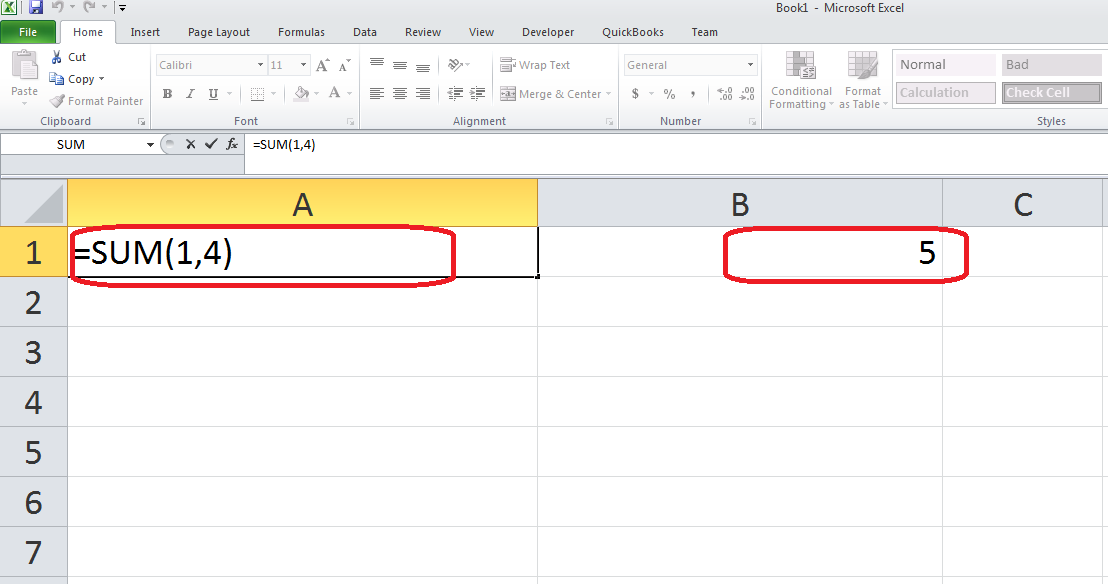Example this will add 1+4 =5. You can add as many numbers you like type comma after each number.

Example 2

For example you want to add data from different cells. A2 to A7

=SUM(A2:A7) this will show you value of 2+2+2+1+1 = 8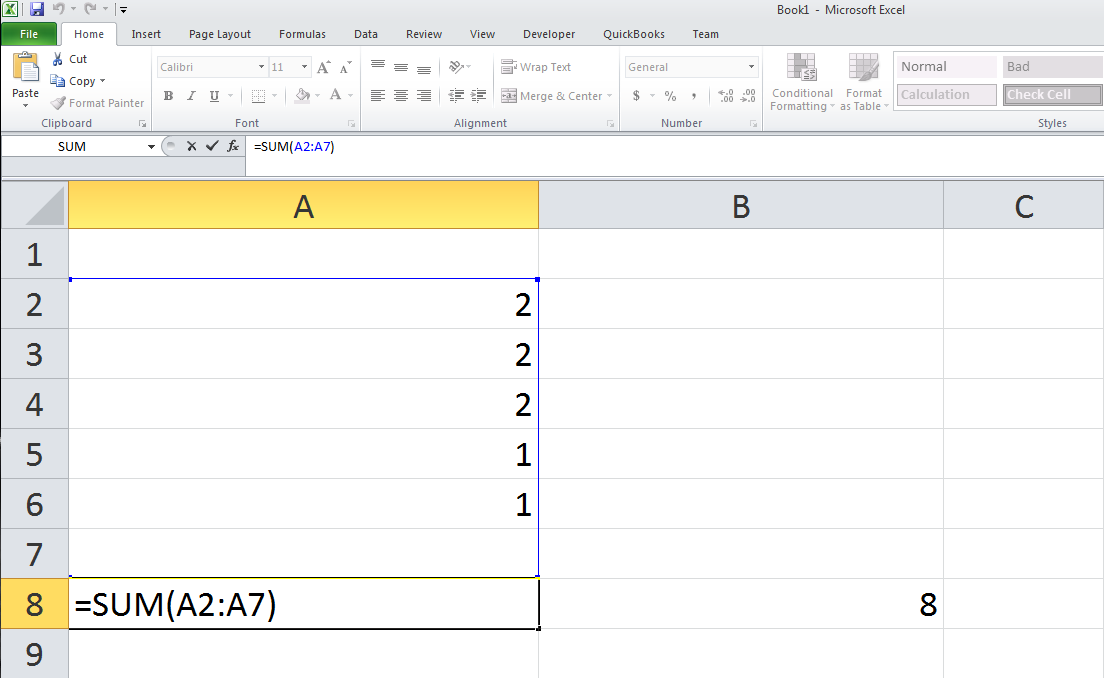In future if you want to add more cell just copy the Cell A8 and move to any new location and change the A7 no to new number.

Example if you want to add value to A8 and A9 column then move formula from A8 to A10 and change A7 to A9.

Example 3

Example you want to add multiple columns with multiple rows in excel spreadsheet.

=SUM(A2:B6)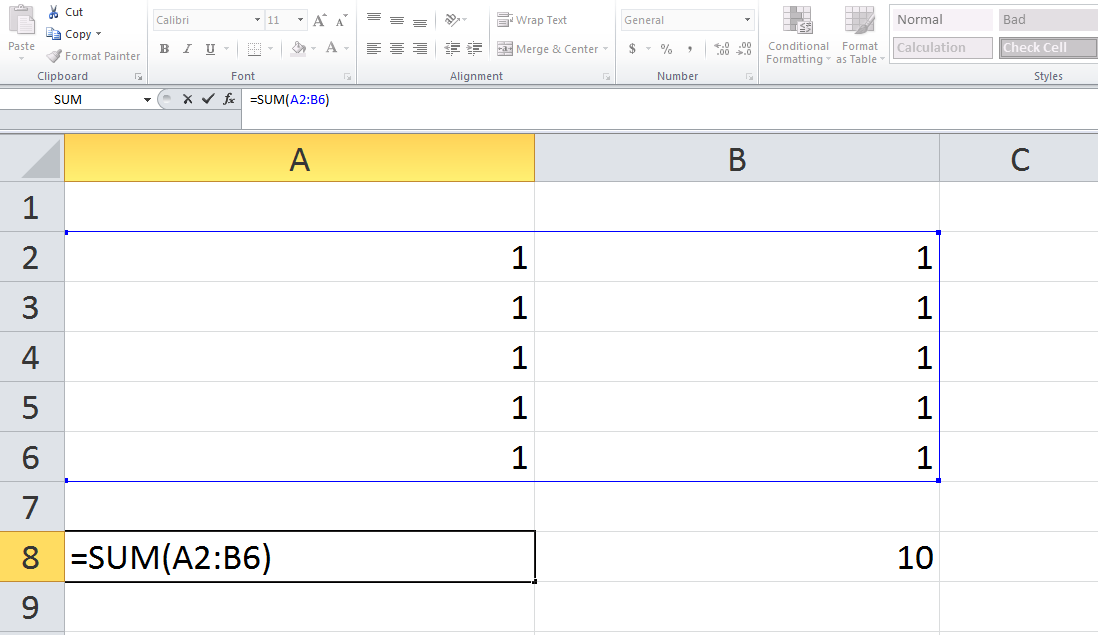This formula will take value from A2 to B6. Column.

Example: 1+1+1+1+1+1+1+1+1+1 = 10

Also it will highlight the field when clicking on the cell with formula. This is very neat feature from Microsoft excel this will help you add two column and multiple rows at same time without using any calculator.

Example 4

Example you want to sum or add value from different cell or random cell selection

=SUM(A2+B2+B3+A4+B5)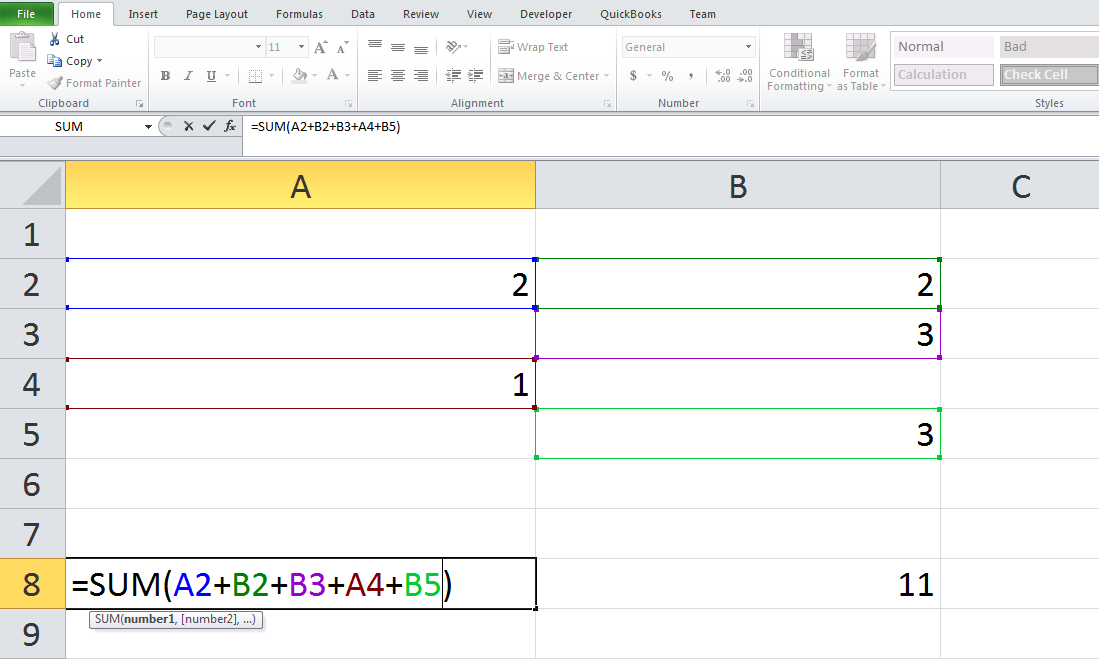You can use any basic math symbols (Plus +, Minus -, Divide /, Multiply *, Percentage %, power of ^). You can mix any type.

Example 5

Multiplying number in excel

Simple different cell multiplication in excel spreadsheet.

Example if you want to multiply different cell you can use formula

=A2*B2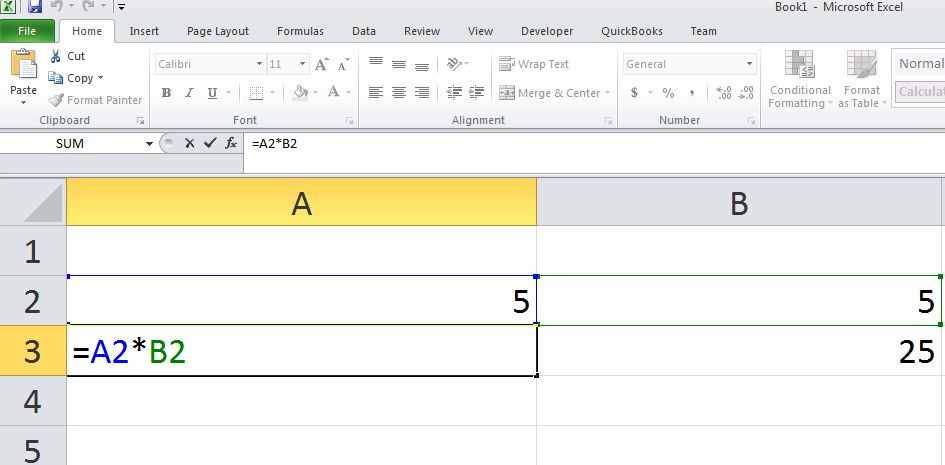In this cell A2 x B2 (5 x 5) = 25. You can add more column by typing * cell number.

Example if you want to multiply cell A2, B2, C2, D2

Excel formula you have to use is =A2* B2* C2* D2

Multiply with simple numbers in excel spreadsheet without using calculator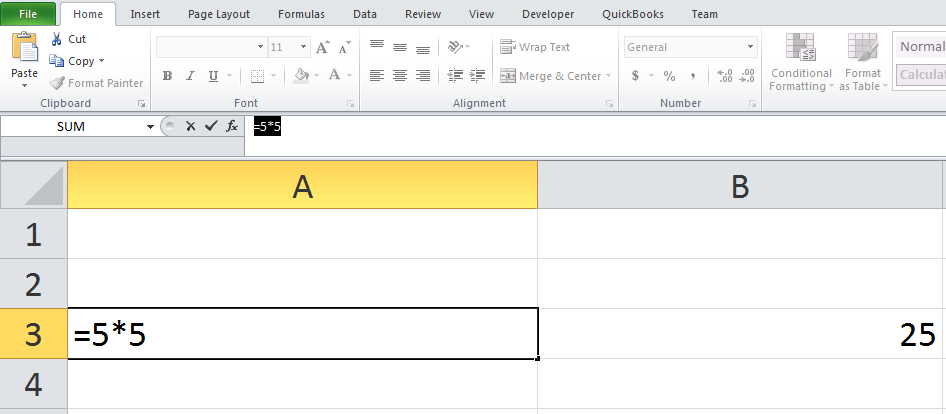This excel formula multiplies 5×5. You don’t have name any cell number. You can add cell number if you want. You can add as many number you want just type * number.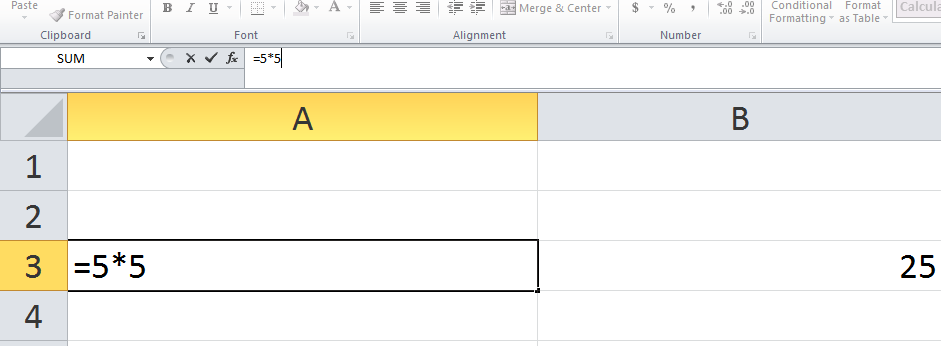Example 6

Dividing numbers in excel using formula

This is simple division . It will divide 10/2 = 5. You can combine multiple cell if you have to.

Dividing different cell in excel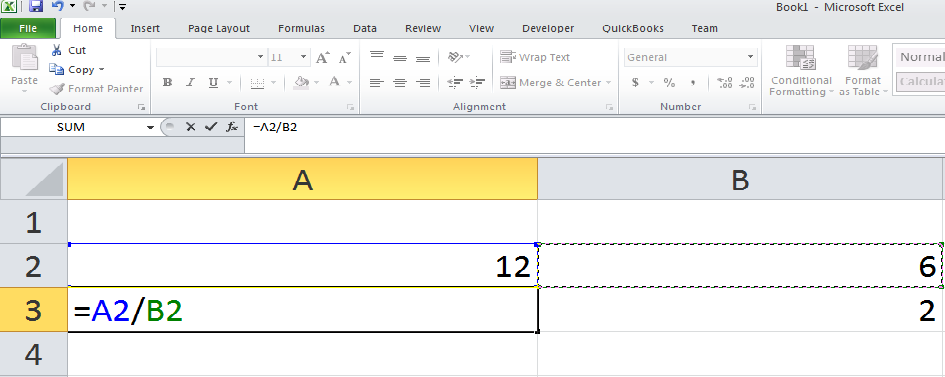This is simple method to divide different cell. This formula is dividing Cell A2/ B2 total of that is 2. Example: 12/6 = 2.

I hope you found this helpful. Let me know if you want me to share any more tips on excel or any other product, I will try to do my best and create a post on that!